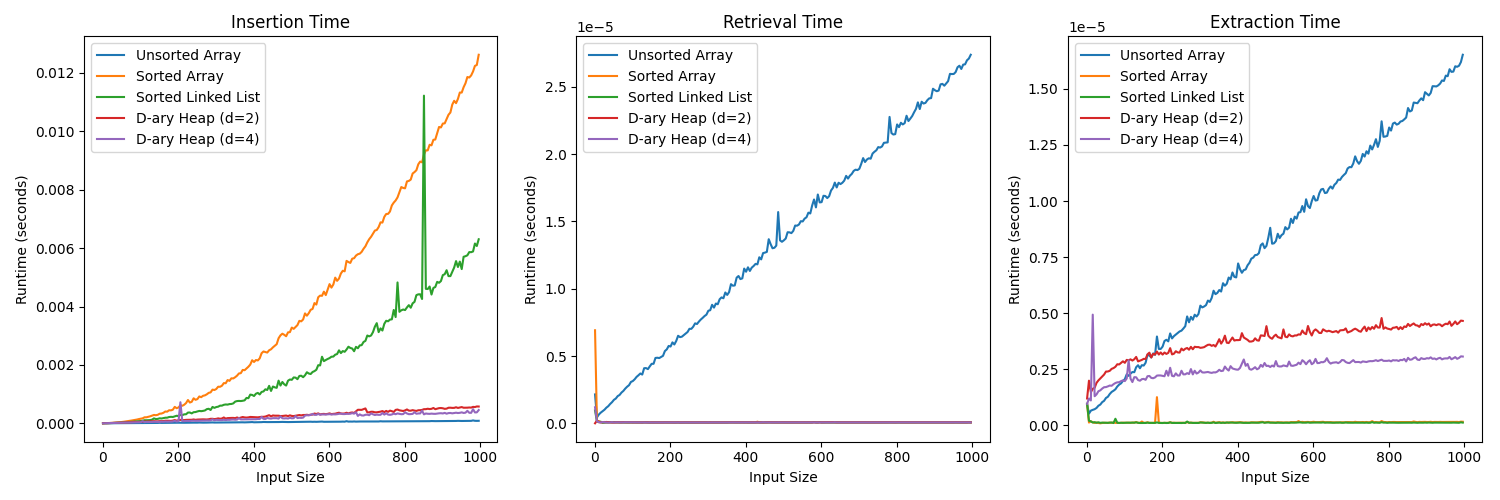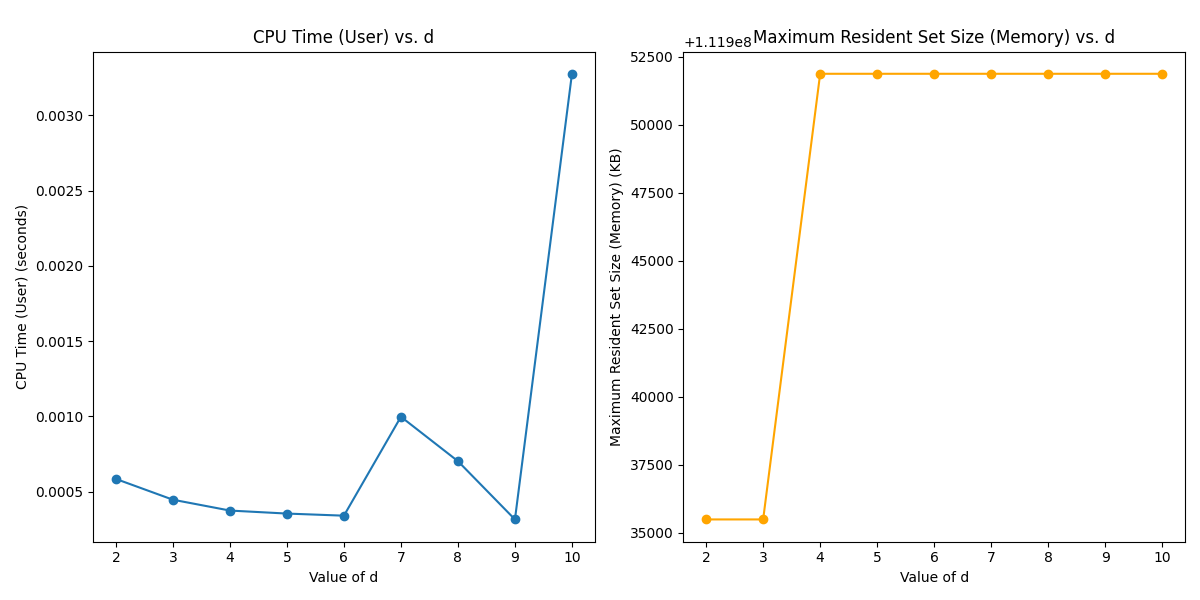# Complexity, Computation and Algorithms

Notes on Complexity theory, Theory of Computation and Algorithms

## Priority Queues

A priority queue is a data structure that maintains a collection of elements, each associated with a priority or key, and provides efficient access to the element with the highest (or lowest) priority. It allows for the insertion and removal of elements based on their priorities, making it a fundamental tool for tasks where items need to be processed in order of importance, such as scheduling tasks, graph algorithms, and simulation. Priority queues can be implemented using various data structures, each with its own trade-offs in terms of time complexity and efficiency.

Priority queues are used in applications requiring efficient management of elements with associated priorities, including scheduling, graph algorithms, data compression, and task prioritization.

1. Insert (Enqueue): This operation allows you to add a new element to the priority queue while ensuring that it is placed in the correct position according to its priority. It should be efficient to insert elements in the order of their priority.

2. Delete (Dequeue): Deleting or dequeuing an element from the priority queue typically removes the element with the highest (or lowest) priority and adjusts the queue to maintain the priority order. This operation should be efficient, and the removed element should be returned.

3. Peek (Front): The peek operation lets you view the element with the highest (or lowest) priority without removing it from the queue. It is essential for checking the next element to be dequeued without altering the queue’s state.

4. IsEmpty: This operation checks whether the priority queue is empty. It returns a boolean value (true if the queue is empty, false if it contains elements). It’s a basic check that helps in controlling the flow of your program.

5. Change Priority: This operation allows you to change the priority of an element in the queue. It is useful when the priority of an element changes during the execution of your program.

### Implementation

1-3 are naive ways to implement a priority queue. 4 is the most efficient way to implement a priority queue, it uses a heap. In priority queues, the most commonly used heap is the binary heap. Binary heaps can be either min-heaps or max-heaps, depending on whether you want the minimum or maximum element to have the highest priority. While insertion is fastest in unsorted arrays, peek and delete are fastest in sorted arrays. Binary heaps are the most efficient way to implement a priority queue, with logarithmic time complexities for insertion and deletion. Binary heaps just outsource the sorting to the insertion algorithm, which is why they are so efficient.

1. Unsorted Array:

• Insert (Enqueue): [$O(1)$] (average case), [$O(n)$] (worst case)
• Delete (Dequeue): [$O(n)$]
• Peek (Front): [$O(n)$]
• IsEmpty: [$O(1)$]
2. Sorted Array:

• Insert (Enqueue): [$O(n)$]
• Delete (Dequeue): [$O(1)$]
• Peek (Front): [$O(1)$]
• IsEmpty: [$O(1)$]

• Insert (Enqueue): [$O(n)$]
• Delete (Dequeue): [$O(1)$]
• Peek (Front): [$O(1)$]
• IsEmpty: [$O(1)$]
4. Binary Heap (Min-Heap or Max-Heap):

• Insert (Enqueue): [$O(\log n)$]
• Delete (Dequeue): [$O(\log n)$]
• Peek (Front): [$O(1)$]
• IsEmpty: [$O(1)$]
5. D-ary heaps can be faster for extraction because with a larger “d,” the elements in each node of the heap are more closely packed together in memory. This can improve cache performance, as accessing elements that are physically close to each other in memory is more cache-friendly. Smaller “d” values may result in elements being more spread out in memory, leading to more cache misses and slower access times. We will show a counter example with resource usages.

p code

### Empirical AnalysisThis image comprises three subplots, collectively providing a comprehensive performance analysis of various priority queue implementations concerning input size and operation runtime. The first subplot demonstrates the insertion time (in seconds) for different priority queue types, such as Unsorted Array, Sorted Array, Sorted Linked List, and D-ary Heaps with varying degrees (d=2 and d=4) as the input size increases. The middle subplot illustrates the average retrieval time per operation, while the rightmost subplot portrays the average extraction time per operation, both showcasing how these times evolve with increasing input size.

You can expect the following trends in the runtime measurements for the priority queue implementations in the generated plots: In the “Insertion Time” subplot, Unsorted Array is likely to have stable times, while Sorted Array and Sorted Linked List may exhibit increasing times due to sorting operations. D-ary Heap (d=2 and d=4) should maintain relatively stable insertion times, with d=4 potentially being slightly faster. In the “Retrieval Time” subplot, Unsorted Array should have stable times, while Sorted Array and Sorted Linked List may show linearly increasing times with input size. D-ary Heap (d=2 and d=4) is expected to perform efficiently with stable retrieval times. In the “Extraction Time” subplot, Unsorted Array should have stable times, while Sorted Array and Sorted Linked List may display linearly increasing times. D-ary Heap (d=2 and d=4) should perform efficiently with stable extraction times. The choice of d in the D-ary Heap may impact performance, with larger d values offering potential slight improvements.

## D-ary heaps vs Binary heaps“Maximum resident set size” (max RSS) is the peak amount of physical memory (RAM) used by a program during its execution, indicating the highest memory usage recorded. It’s a measure of a program’s memory efficiency and is often used for memory optimization and profiling. We see for some reason, at [$d=6, d=9$] we find the lowest usages via CPU times.

Source: https://theoryofcomputation.xyz/blog/priority-queue/, 0001-01-01